ConceptDraw
DIAGRAM 14

# Venn Diagram Template

It's impossible to overestimate the usefulness and convenience of using the ready templates when you create your own diagrams and charts. And Venn Diagrams are not exception.

ConceptDraw DIAGRAM vector diagramming and drawing software presents the Business Diagrams solution from Management area which offers a set of Venn Diagram templates and samples. Use the suitable Venn Diagram Template to create your own Venn Diagram of any complexity.

Look at this variety of Venn Diagram templates!

All they are included in powerful Business Diagrams Solution for ConceptDraw Solution Park. You can find each of them in ConceptDraw STORE and click to open in ConceptDraw DIAGRAM.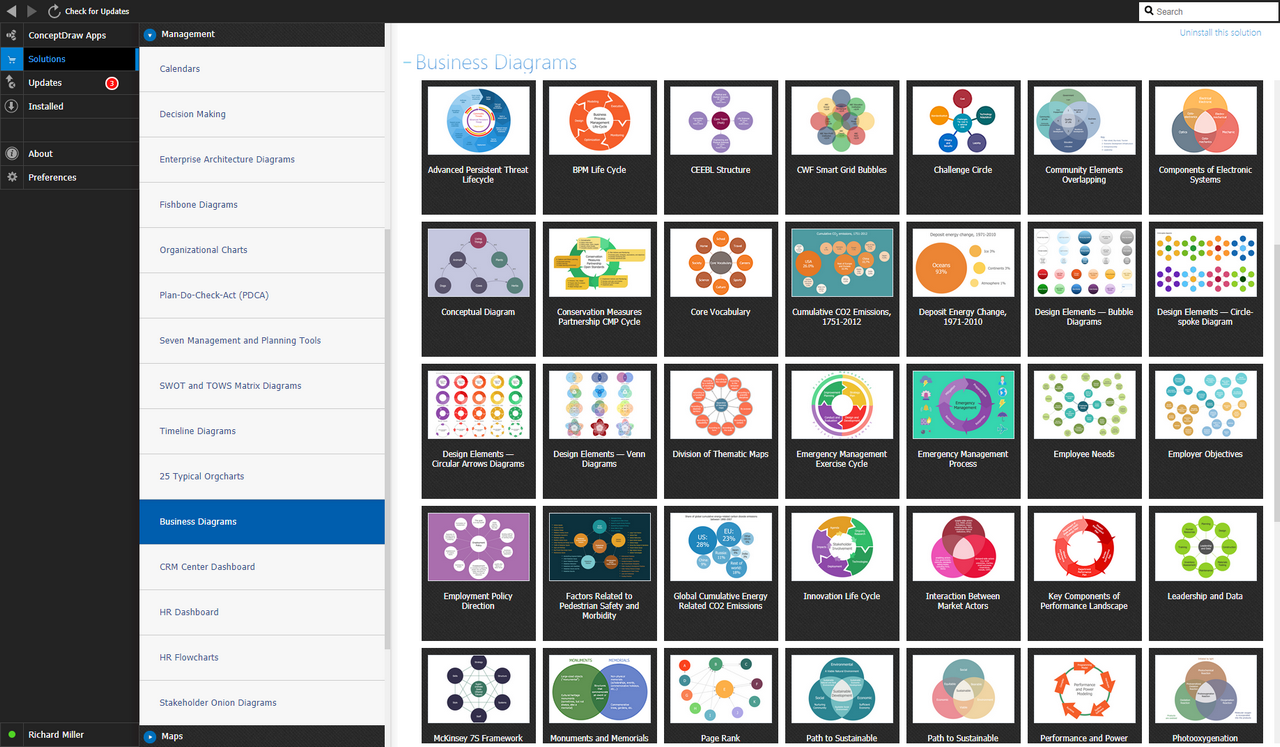Example 1. Business Diagrams Solution in ConceptDraw STORE

Use of Venn Diagram template is a modern way of easy drawing professional looking Venn Diagrams. Try and verify this!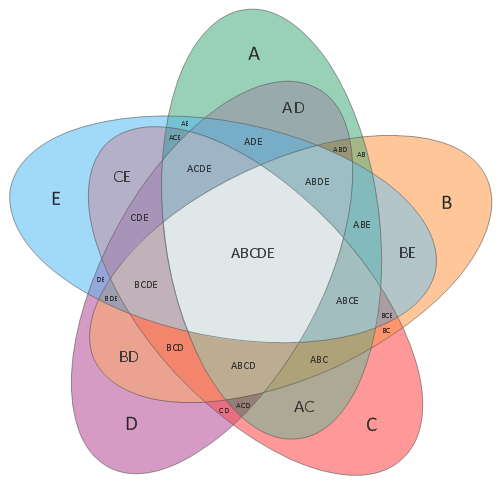Example 2. 5-Set Venn Diagram Template

This example illustrates the 5-Set Venn Diagram Template which was designed for ConceptDraw DIAGRAM users and can be opened for filling from the Business Diagrams solution section in ConceptDraw STORE. Now, thanks to ConceptDraw DIAGRAM designing the Venn Diagram of any complexity on the base of template will take only few minutes.

Use Business Diagrams solution to effectively design your own Venn Diagrams and then successfully use them.

All source documents are vector graphic documents. They are available for reviewing, modifying, or converting to a variety of formats (PDF file, MS PowerPoint, MS Visio, and many other graphic formats) from the ConceptDraw STORE. The Business Diagrams Solution is available for ConceptDraw DIAGRAM users.

### Venn Diagram Examples for Problem Solving.Venn Diagram as a Truth Table →

Venn diagrams are illustrations used in the branch of mathematics known as set theory. They show the mathematical or logical relationship between different groups of things (sets). A Venn diagram shows all the possible logical relations between the sets.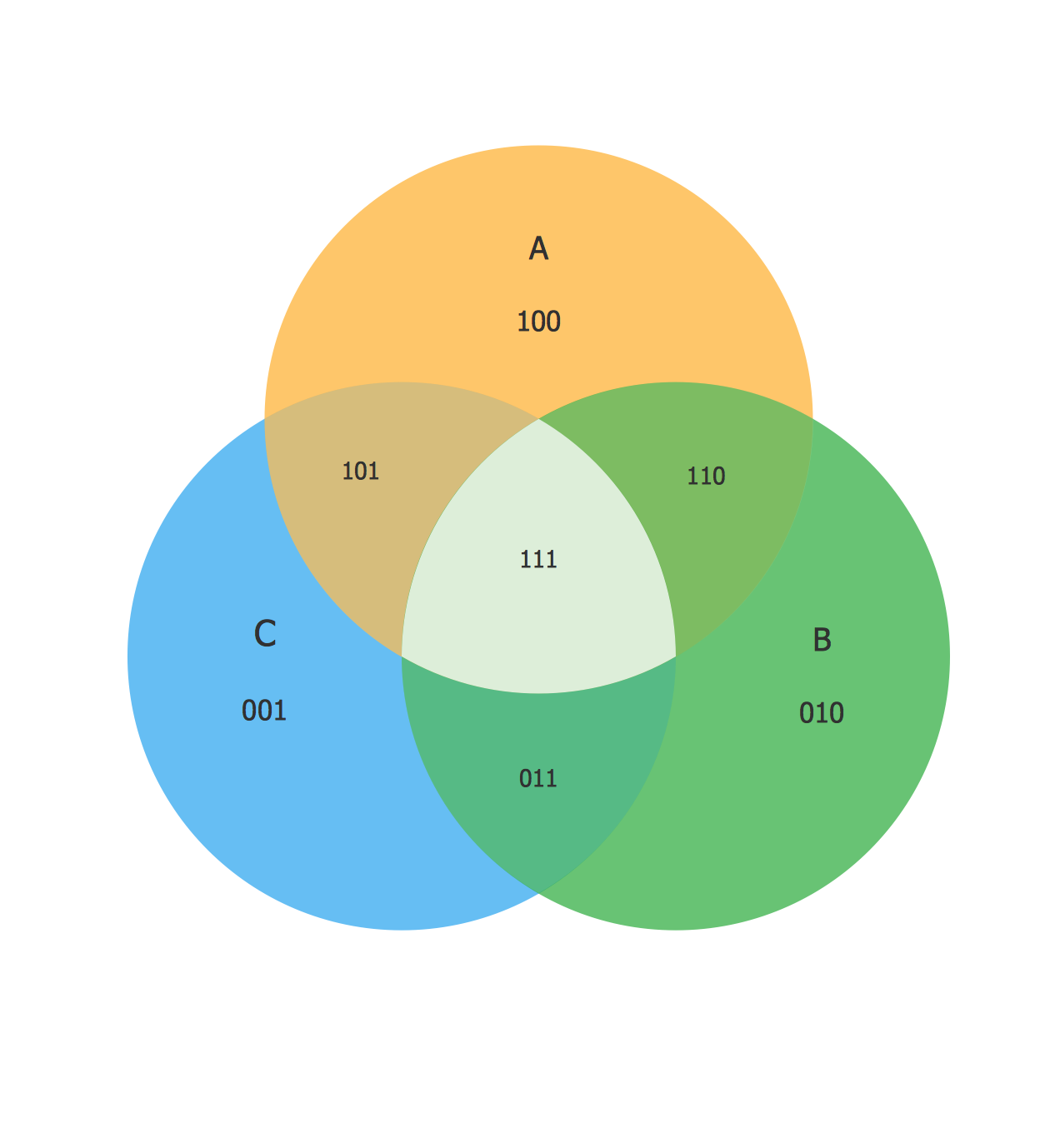Picture: Venn Diagram Examples for Problem Solving.Venn Diagram as a Truth Table
Related Solution:

### Pyramid Diagram →

pyramida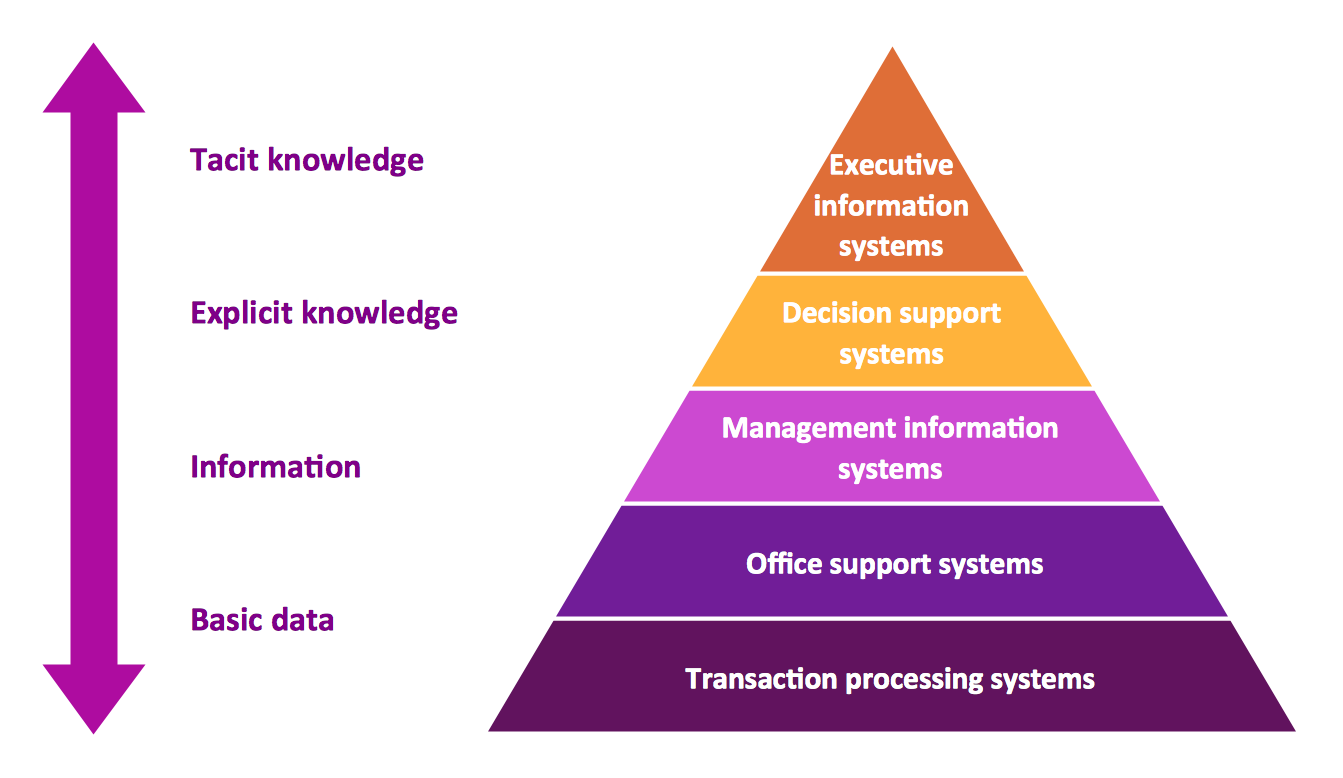Picture: Pyramid Diagram
Related Solution:

### Multi Layer Venn Diagram. Venn Diagram Example →

To visualize the relationships between subsets of the universal set you can use Venn diagrams. To construct one, you should divide the plane into a number of cells using n figures. Each figure in the chart represents a single set of, and n is the number of represented sets. Splitting is done in a way that there is one and only one cell for any set of these figures, the points of which belong to all the figures from the set and do not belong to others. The plane on which the figures are represented, is the universal set U. Thus, the point which does not belong to any of the figures, belongs only to U.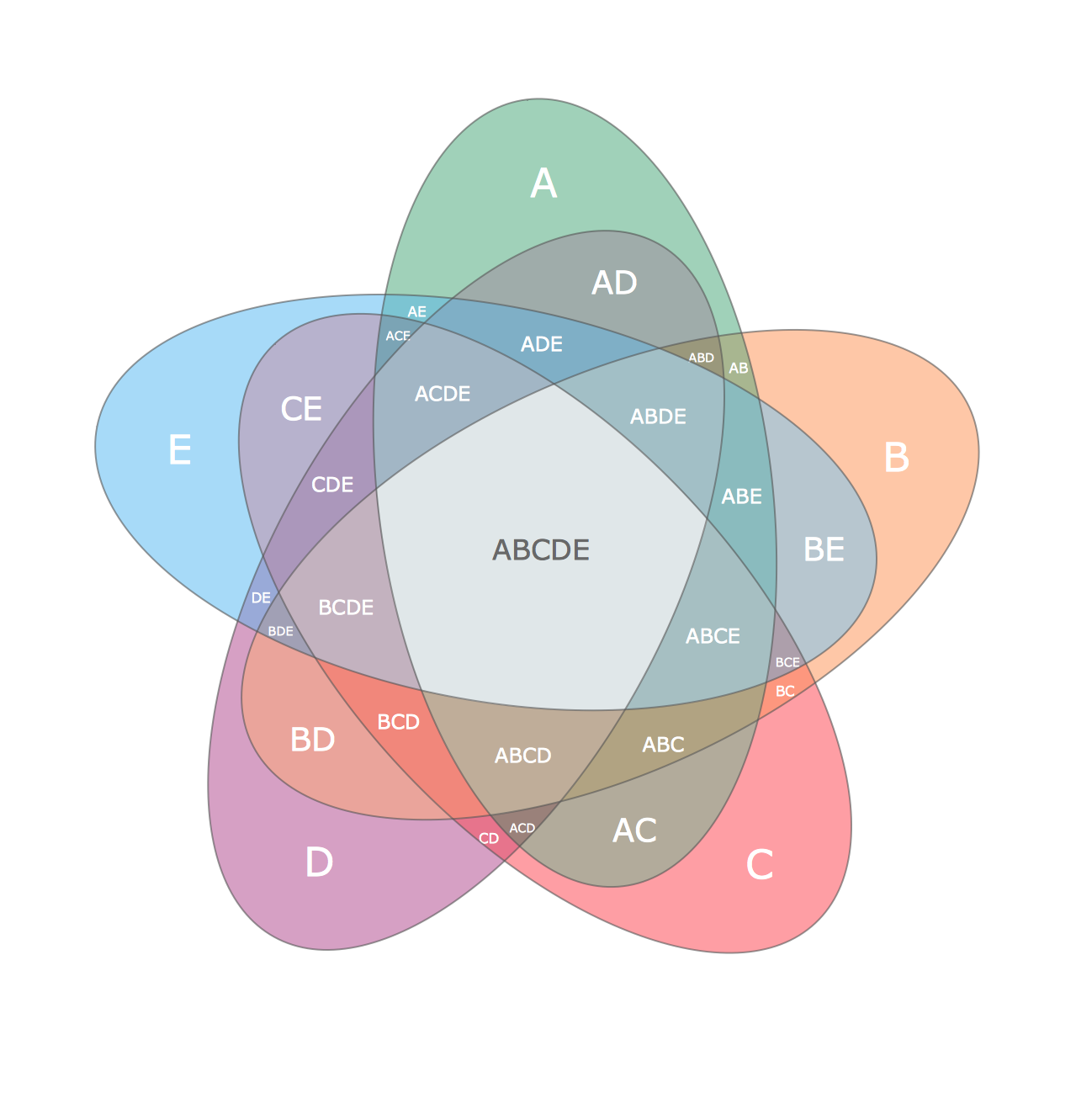Picture: Multi Layer Venn Diagram. Venn Diagram Example
Related Solution:
ConceptDraw
DIAGRAM 14